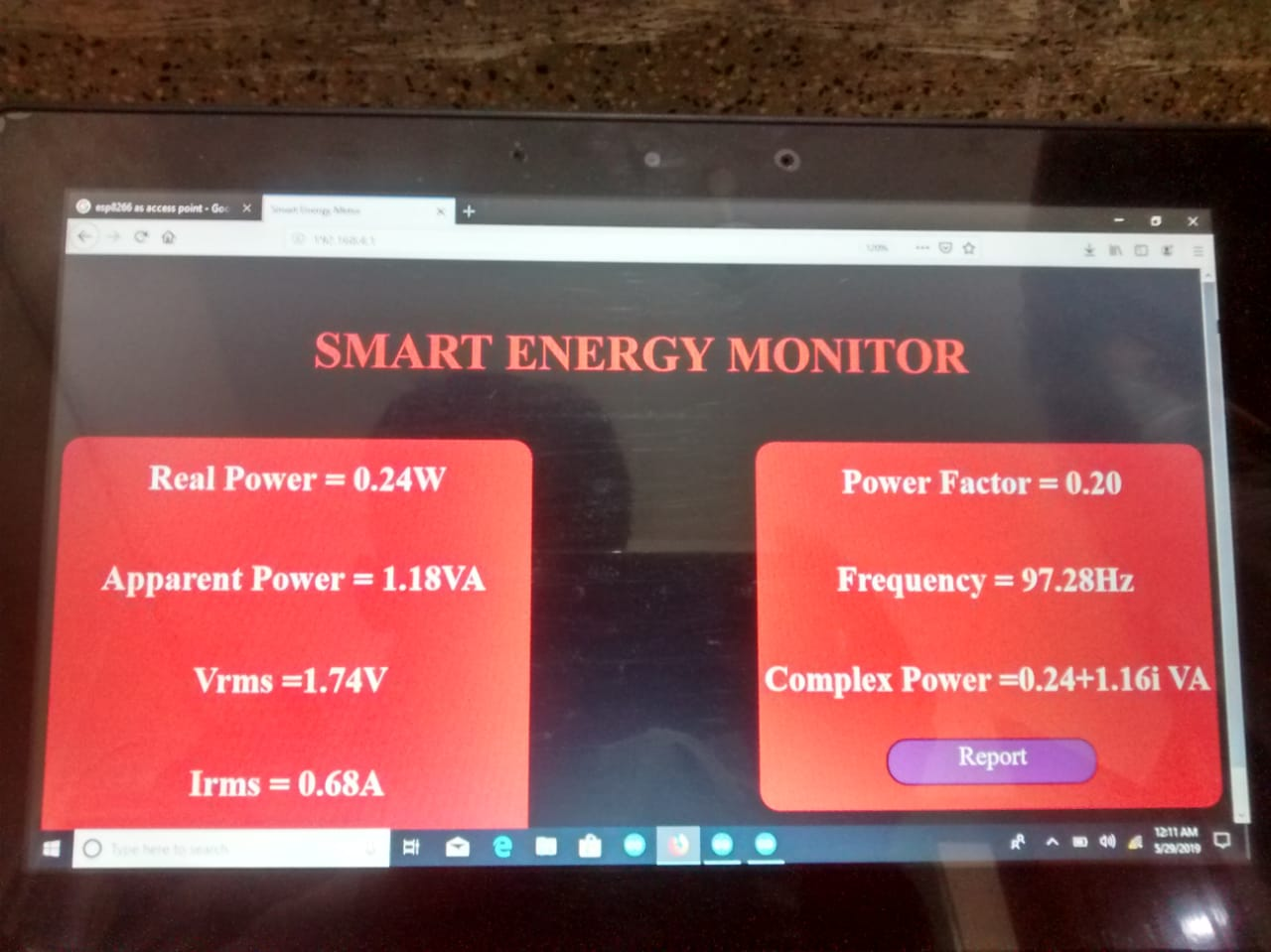Arduino

# SMART ENERGY METERJune 13, 2019 by Mohammad Misbah
Sharewifi enabled smart energy meter based on arduino that shows electrical quantites of an appliance on a web server.

## Hardware

 1 Arduino UNO 1 Node MCU 1 ACS 712 1 ZMPT 101 B 1 jumper wires 1 breadboard

## Software

 1 arduino ide

### INTRODUCTION:

Wifi enabled energy meter based on arduino that shows electrical quantites for any appliance on a web server.

Libraries:

1. Emonlib - download it from https://github.com/openenergymonitor/EmonLib . Then install it in libraries folder.

2. ArduinoJSON -

Open the Arduino Library Manager

Search for â€œArduinoJsonâ€

Select the version: 5.13.5

Click install.

see video ---  https://youtu.be/GUTpaY1YaXo

3.Software Serial - Arduino has a Library of Software Serial in it. If you canâ€™t find its library then you should download the Software Serial Library.  https://www.arduino.cc/en/Reference/softwareSerial

``````// this code is uploaded to arduino.
//Remember to install all included libraries first.

#include <EmonLib.h>            // Include Emon Library

#include <SoftwareSerial.h>    //This library will do serial communication b/w arduino and nodemcu.

#include<ArduinoJson.h>       //This library is used to transfer data from arduino to nodemcu.

EnergyMonitor emon1;          // Create an instance

SoftwareSerial s(5,6);       //We named serialport as "s" in which pin 5 is RX(receiving) and pin 6 is TX(transmitting).

void setup()
{
Serial.begin(9600);        //Serial monitor begin at 9600 baud rate.
s.begin(9600);             //Serial Port "s" begin at 9600 baud rate.

emon1.voltage(2, 178, 1.7);  // Voltage: input pin, calibration, phase_shift
emon1.current(1, 5);       // Current: input pin, calibration.60
}
StaticJsonBuffer<1000> jsonBuffer;     //here json is used to transmit data from arduino to nodemcu.
JsonObject& root = jsonBuffer.createObject();   //json object created to store data.

void loop()
{

emon1.calcVI(20,2000);         // Calculate all. No.of wavelengths, time-out
emon1.serialprint();           // Print out all variables
emon1.calcF(50);               // 50 zero crossings
float fre = emon1.frequency;  // calculate frequency.
float freq = fre + 5;

Serial.print("Frequency: ");   //print Frequency in serial window.
Serial.println(freq);          //print value of frequency.

float real=emon1.realPower;    //calculate real power/
float apparent=emon1.apparentPower;  //calculate apparent power.

float sq_Ap = apparent*apparent;
float sq_Rp = real*real;
float diff_of_sq = sq_Ap - sq_Rp;
Serial.print("apparent squared: ");   //print Frequency in serial window.
Serial.println(sq_Ap);
Serial.print("real squared: ");   //print Frequency in serial window.
Serial.println(sq_Rp);
Serial.print("diff of sq: ");   //print Frequency in serial window.
Serial.println(diff_of_sq);
float reactive=sqrt(diff_of_sq);
Serial.print("reactive: ");   //print Frequency in serial window.
Serial.println(reactive);

delay(1000);
//float reactive=sqrt(diff_of_sq);
root["RP"] = emon1.realPower;   //calculated real power is stored in json object "RP".
root["AP"] = emon1.apparentPower;  //calculated apparent power is stored in json object "AP".
root["VRMS"] = emon1.Vrms;    //calculated Vrms is stored in json object "VRMS".
root["IRMS"] = emon1.Irms;    //calculated Irms is stored in json object "IRMS".
root["PF"] = emon1.powerFactor;  //calculated power factor is stored in json object "PF".
root["FREQ"] = freq;          //calculated frequency is stored in json object "FREQ".
root["reactive"] = reactive;  //calculated reactive power is stored in json object "reactive".

if(s.available()>0)    //if received anything from nodemcu through serial port then print all root objects to serial port.
{
root.printTo(s);
}
}``````

Connections:

For current sensing connect acs712 in series with your appliance.

Current Sensor --------------------- > Arduino

Vcc ---------------------------------> 5v

GND ---------------------------------> GND

OUT --------------------------------> A1 (this can be of any analog pin of your choice)

For Voltage Sensing connect ZMPT 101B in parallel with appliance. Connections are as follows:

voltage sensor ------------> Arduino

Vcc ---------------------> 5v

GND(both) -------------- > GND

OUT -----------------------> A2 (this can be of any analog pin of your choice)

For Serial Communication between arduino and nodemcu the connections are as follows:

Arduino ---------------- > Node MCU

5 (Rx) -------------------- > D5 (Tx)

6 (Tx) -------------------- > D6 (Rx)